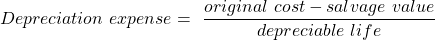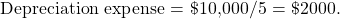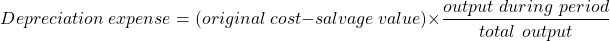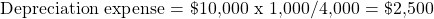Concept 53: Depreciation Methods | IFT World
101 Concepts for the Level I Exam

# Concept 53: Depreciation MethodsDepreciation methods are:

• Straight line – The cost of an asset is evenly distributed over the asset’s useful life.
• Accelerated – A higher depreciation expense is recorded in the early years and lower depreciation expense is recorded in the later years of an asset’s life. Double declining balance (DDB) is one example of an accelerated depreciation method.
• Units of production – Cost allocated is based on the actual use of an asset in a particular period.

Straight line depreciation expense = depreciable cost / estimated useful life

DDB depreciation expense = 2 x straight-line rate x beginning book value

Units of production depreciation expense per unit = depreciable cost / useful life in units

Carrying amount = historical cost – accumulated depreciation
Depreciable cost = historical cost – estimated residual value

An analyst gathered the following information about an equipment’s expected production life and use. The equipment was purchased for \$10,000 and is expected to have 0 salvage value at the end of its useful life.

 Year 1 Year 2 Year 3 Year 4 Year 5 Units produced 1,000 1,100 900 500 500

Calculate the Year 1 depreciation expense under the units-of-production method and the straight-line method.

Solution:

Straight line method:Units of production method:** IFT Website Un-available on Monday 2-October-2023, 0700-1200 GMT **
This is default text for notification bar